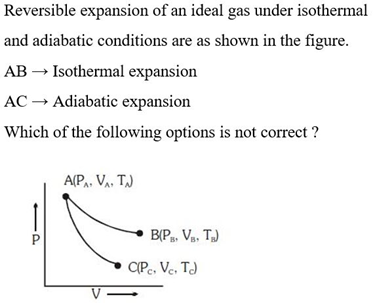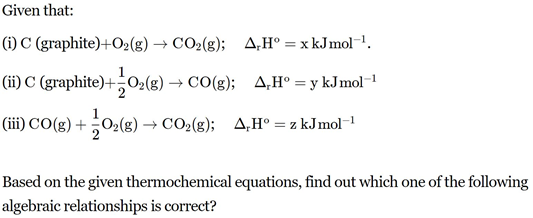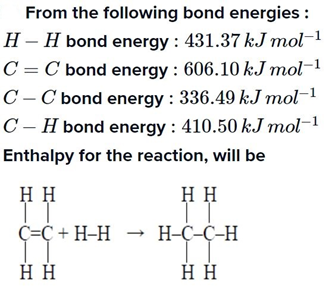# NEET Thermodynamics Questions : NEET Previous Year questions

1. Which of the following are not state functions?                                 (2008)

(I) q + w                               (II) q

(III) w                                  (IV) H – TS

(a) (I), (II)
and (III)            (b) (II) and (III)

(c) (I) and
(IV)                   (d) (II), (III)
and (IV)

Chemistry Notes

Physics Notes

Biology Notes

(a) q = 0, ΔT = 0 and w = 0

(b) q = 0, ΔT < 0 and w > 0

(c) q < 0, ΔT = 0 and w = 0

(d) q > 0, ΔT > 0 and w > 0

5. Under isothermal conditions, a gas at 300 K expands from 0.1 L to 0.25 L against a constant external pressure of 2 bar. The work done by the gas is

[Given that 1 L bar = 100 J]                                                        (NEET 2019)

(a) 30 J                            (b) –30 J

(c) 5 kJ                            (d) 25 J

6.(NEET 2019)

(b) TA = TB

(d) TC = TA7. An ideal gas expands isothermally from 10–3 m3 to 10–2 m3 at 300 K against a constant pressure of 105 N m–2. The work done on the gas is  (NEET 2019)

(a) +270 kJ                 (b) –900 J

(c) +900 kJ                 (d) –900 kJ

8. A gas is allowed to expand in a well-insulated container against a constant external pressure of 2.5 atm from an initial volume of 2.50 L to a final volume of 4.50 L. The change in internal energy ΔU of the gas in joules will be                                                (NEET 2017)

(a) –500 J                       (b) –505 J

(c) +505 J                       (d) 1136.25 J

9. Equal volumes of two monatomic gases, A and B at same temperature and pressure are mixed. The ratio of specific heats (CP/CV) of the mixture will be                   (2012)

(a) 0.83                             (b) 1.50

(c) 3.3                               (d) 1.67

10. Which of the following is correct option for free expansion of an ideal gas under adiabatic condition?                                                     (2011)

(a) q = 0, ΔT ≠ 0, w = 0                      (b) q ≠ 0, ΔT = 0, w = 0

(c) q = 0, ΔT = 0, w = 0                      (d) q = 0, ΔT < 0, w ≠ 0

11. Three moles of an ideal gas expanded spontaneously into vacuum. The work done will be                                                              (Mains 2010)

(a) Infinite              (b) 3 Joules

(c) 9 Joules            (d) zero.

12. Assume each reaction is carried out in an open container. For which reaction will ΔH = ΔE?                                                                (2006)

(a) 2CO(g)+ O2(g)→ 2CO2(g)

(b) H2(g)+ Br2(g)) →2HBr(g)

(c) C(s) + 2H2O(g) → 2H2(g)+ CO2(g)

(d) PCl5(g) →   PCl3(g)+ Cl2(g)

23.(NEET 2013)

(a) z = x + y                               (b) x = y + z

(c) y = 2z – x                            (d) x = y – z

24. Standard enthalpy of vaporization  ΔvapH° for water at 100°C is 40.66 kJ mol–1. The internal energy of vaporization of water at 100°C (in kJ mol–1) is             (NEET 2013)

(a) +37.56                 (b) – 43.76

(c) + 43.76                (d) + 40.66

25. Consider the following processes:                               (Mains 2011)

ΔH (kJ/mol)

1/2A → B                        +150

3B → 2C + D                     125

E + A → 2D                      +350

For B + D → E + 2C, ΔH will be

(a) 525 kJ/mol              (b) –175 kJ/mol

(c) –325 kJ/mol           (d) 325 kJ/mol

26. The following two reactions are known                       (Mains 2010)

Fe2O3(s) + 3CO(g) → 2Fe(s) + 3CO2(g); ΔH = –26.8 kJ

Fe O(s) + CO(g) → Fe (s) + CO2(g); ΔH = – 16.5 kJ

The value of ΔH for the following reaction

Fe2O3(s) + CO(g) → 2FeO(s) + CO2(g) is

(a) + 10.3 kJ                    (b) – 43.3 kJ

(c) – 10.3 kJ                    (d) + 6.2 kJ

34. The bond dissociation energies of X2 , Y2 and XY are in the ratio of 1 : 0.5 : 1. ΔH for the formation of XY is –200 kJ mol The bond dissociation energy of X2 will be  (NEET 2018)

(a) 200 kJ mol–1               (b) 100 kJ mol–1

(c) 800 kJ mol–1               (d) 400 kJ mol–1

35. The heat of combustion of carbon to CO2 is –393.5 kJ/mol. The heat released upon formation of 35.2 g of CO2 from carbon and oxygen gas is           (2015)

(a) + 315 kJ/mole                           (b) – 630 kJ/mole

(c) – 3.15 kJ/mole                          (d) – 315 kJ/mole

36. When 5 liters of a gas mixture of methane and propane is perfectly combusted at 0°C and 1 atmosphere, 16 litres of oxygen at the same temperature and pressure is consumed. The amount of heat released from this combustion in

kJ (ΔHcomb (CH4) = 890 kJ mol–1,

ΔHcomb. (C3H8) = 2220 kJ mol–1) is                                   (NEET 2013)

(a) 38          (b) 317       (c) 477          (d) 32

37. Enthalpy change for the reaction, 4H(g) → 2H2(g) is –869.6 kJ

The dissociation energy of H – H bond is                                    (2011)

(a) – 434.8 kJ                (b) – 869.6 kJ

(c) + 434.8 kJ               (d) + 217.4 kJ

38.(2009)

(a) – 243.6 kJ mol–1              (b) – 120.0 kJ mol–1

(c) 553.0 kJ mol–1                         (d) 1523.6 kJ mol–1